Question

# Write a program in C++ language that finds the largest number in an array of positive...

Write a program in C++ language that finds the largest number in an array of positive numbers using recursion. Your program should have a function called array_max that should pass in an int array, and an int for the size of the array, respectively, and should return an int value. As an example, given an array of size 10 with contents: {10, 9, 6, 1, 2, 4, 16, 8, 7, 5} The output should be: The largest number in the array is 16 The function should return -1 if the input is invalid. Place the array_max's function prototype in array_max.hpp and its implementation in array_max.cpp. The main function already contains some code, but you need to complete the requirements that is described inside the file.

CODE WITH EXPLANATION IS BELOW:-

#include <iostream>

using namespace std;

int array_max(int arr[], int n)

{ //here let's observe how this code works.

// let's take an array of size 3 arr={2,1,3}

// when we will call the function with araay_max(arr,3)

// n==1 will be false for the first recursion

// 1.therefore it will return max(arr[3-1],array_max(arr,2)), arr[3-1] = arr = 3

//and again array_max will go in another recusrsion and check

//2.and therefore it will return max(arr[2-1],array_max(arr,1)), arr[2-1] = arr = 1

//and again array_max will go in another recusrsion and check

// but this arr has only one element which is 2 therefore it return 2 to step 2

// and will compare between 2 and 1 and return 2 to step 1

// and there 2 and 3 will be compareed and 3 will be returned

// so basically the comarision is 1,2 then 2,3

if (n == 1)

return arr;

return max(arr[n-1], array_max(arr, n-1));

}

int main()

{

int arr[] = {10, 9, 6, 1, 2, 4, 16, 8, 7, 5}; // this the test array of size ten

int n = 10;

cout << array_max(arr, n); // printing what is returned by the araay_max

return 0;

}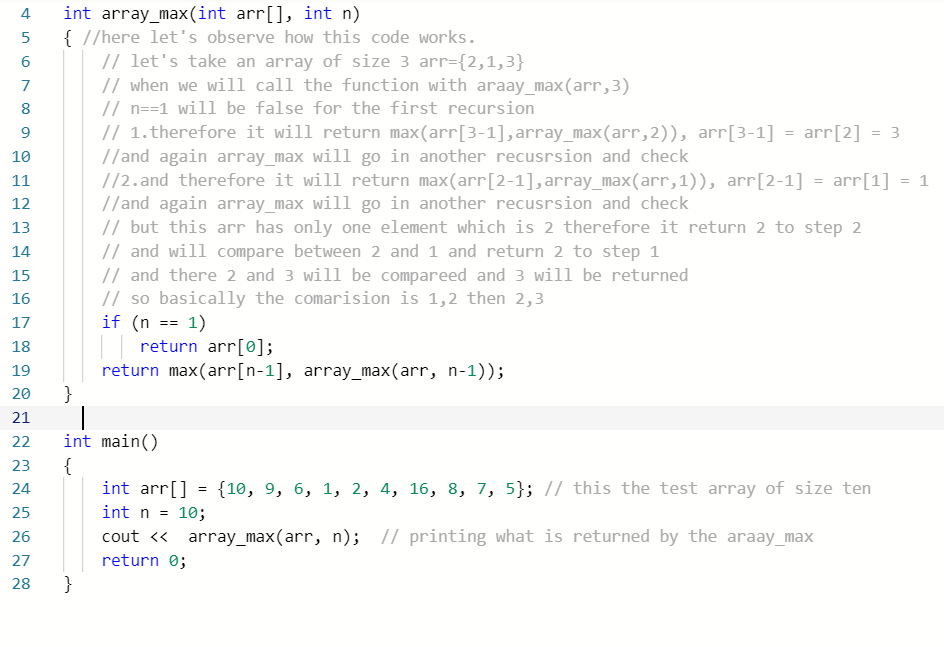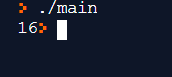#### Earn Coins

Coins can be redeemed for fabulous gifts.

Similar Homework Help Questions
• ### In C language Write a program that includes a function search() that finds the index of...

In C language Write a program that includes a function search() that finds the index of the first element of an input array that contains the value specified. n is the size of the array. If no element of the array contains the value, then the function should return -1. The program takes an int array, the number of elements in the array, and the value that it searches for. The main function takes input, calls the search()function, and displays...

• ### - Write a program that performs several operations on an array of positive ints. The program...

- Write a program that performs several operations on an array of positive ints. The program should prompt the user for the size of the array (validate the size) and dynamically allocate it. Allow the user to enter the values, do not accept negative values in the array. - Your program should then define the functions described below, call them in order, and display the results of each operation: a) reverse: This function accepts a pointer to an int array...

• ### (C++ program )Write a function that accepts an int array and the array’s size as arguments. The function should create a new array that is one element larger than the argument array. The first element...

(C++ program )Write a function that accepts an int array and the array’s size as arguments. The function should create a new array that is one element larger than the argument array. The first element of the new array should be set to 0. Element 0 of the argument array should be copied to the element 1 of the new array. Element 1 of the argument array should be copied to element 2 of the new array, and so forth....

• ### Write a C++ program that demonstrates use of programmer-defined data structures (structs), an array of structs, passing...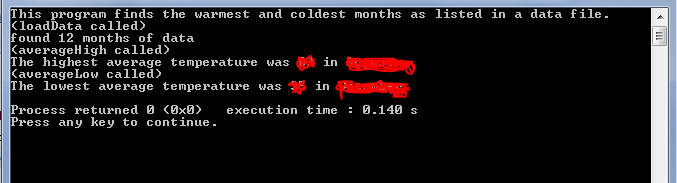Write a C++ program that demonstrates use of programmer-defined data structures (structs), an array of structs, passing an array of structs to a function, and returning a struct as the value of a function. A function in the program should read several rows of data from a text file. (The data file should accompany this assignment.) Each row of the file contains a month name, a high temperature, and a low temperature. The main program should call another function which...

• ### please use c ++ language and write the comment too. thanks Pointer Arithmetic Write a function...

please use c ++ language and write the comment too. thanks Pointer Arithmetic Write a function that passes an array address to a function as a pointer. Using pointer arithmetic, the function determines the average, high, and low value of the array. Your function should have this header: void pointerArithmetic(int *array, int size, double &average, int &high, int &low) Parameter size is the number of elements in array. You may not use the subscript notation [] inside your function –...

• ### Write a C program convert.c that converts each number in an array by the sum of...

Write a C program convert.c that converts each number in an array by the sum of that number plus 6 modulus 10. A sample input/output: Enter the length of the array: 5 Enter the elements of the array: 3 928 4 14 77 Output: 9 4 0 0 3 The program should include the following function: void convert(int *a1, int n, int *a2) The function converts every element in array a1 of length n to an output array a2. The...

• ### c++ program8. Array/File Functions Write a function named arrayToFile. The function should accept three arguments:...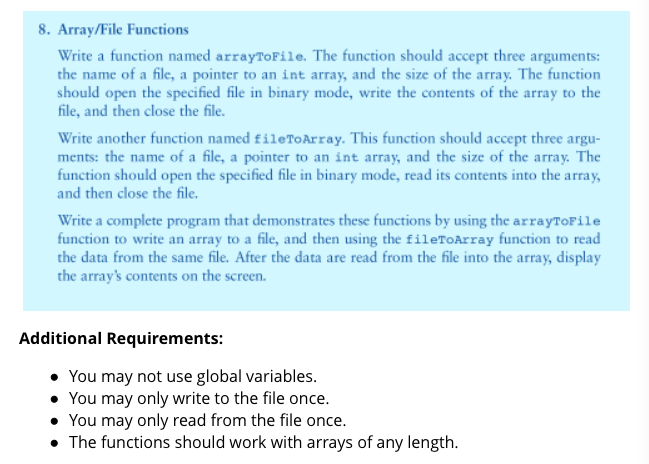c++ program8. Array/File Functions Write a function named arrayToFile. The function should accept three arguments: the name of a file, a pointer to an int array, and the size of the array. The function should open the specified file in binary mode, write the contents of the array to the file, and then close the file. Write another function named fileToArray. This function should accept three argu- ments: the name of a file, a pointer to an int array, and the size...

• ### Write a C program Design a program that uses an array to store 10 randomly generated...

Write a C program Design a program that uses an array to store 10 randomly generated integer numbers in the range from 1 to 50. The program should first generate random numbers and save these numbers into the array. It will then provide the following menu options to the user: Display 10 random numbers stored in the array Compute and display the largest number in the array Compute and display the average value of all numbers Exit The options 2...

• ### (C++) Write a function that accepts an int array and the array’s size as arguments. The function...

(C++)Write a function that accepts an int array and the array’s size as arguments.The function should create a new array that is twice the size of the argument array.The function should copy the contents of the argument array to the new array, and initialize the unused elements of the second array with 0.The function should return a pointer to the new array.Demonstrate the function by using it in a main program that reads an integer N (that is not more...

• ### Write a C++ function, lastLargestIndex that takes as parameters an int array and its size and...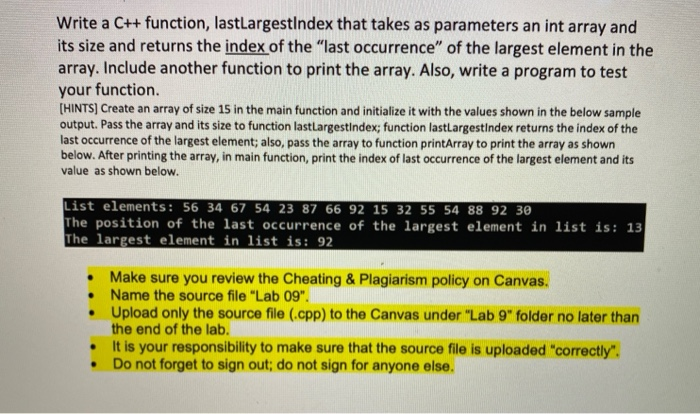Write a C++ function, lastLargestIndex that takes as parameters an int array and its size and returns the index of the "last occurrence" of the largest element in the array. Include another function to print the array. Also, write a program to test your function. [HINTS) Create an array of size 15 in the main function and initialize it with the values shown in the below sample output. Pass the array and its size to function lastLargestindex; function lastLargestindex returns...